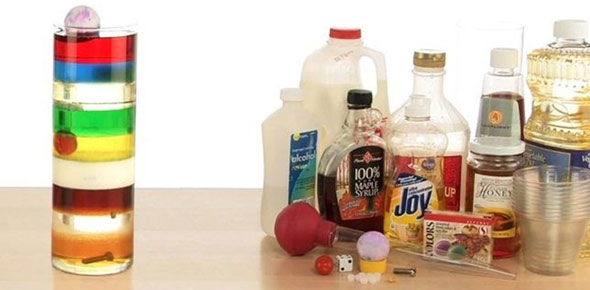# Density Column Quiz

10 Questions | Total Attempts: 141SettingsRelated Topics
• 1.
What are the minimum number of layers in your density column?
• A.

Two

• B.

Three

• C.

Five

• 2.
The most dense liquids goes where?
• A.

Top

• B.

Bottom

• C.

Middle

• 3.
True or False. You can shake a density column and all the layers will go back to where they previoulsy were.
• A.

True

• B.

False

• 4.
Can the liquids touch the sides as your pour them?
• A.

Yes

• B.

No

• 5.
What would happen if you poured a more dense liquid on a less dense one?
• A.

Your density column would be normal

• B.

Your density column would be ruined, all the liquids would mix together

• 6.
What is density?
• A.

Mass per unit of volume

• B.

Mass times velocity

• 7.
Almost any liquids can be used in a density column. What liquid should you not use?
• A.

Water

• B.

Liquid Nitrogen

• C.

Dish soap

• 8.
What liquid would you most likely use as your first layer? (most dense)
• A.

Water

• B.

Corn Starch

• C.

Molasses

• D.

Alcohol

• 9.
What liquid would you use as your final layer? (least dense)
• A.

Cement Mix

• B.

Alcohol

• C.

Water

• D.

Honey

• 10.
Water and oil are hard to mix. Why do you think this is so?
• A.

They are different densities.

• B.

They hate each other.

• C.

Cheese.